Racer

The first racer run at a speed 20 m/s and is 750 meters before the finish and ahead 79 meters before second competitor.

At what speed must run the second racer to catch first racer at the finish line?

Result

v2 =  22.11 m/s

Solution:Leave us a comment of this math problem and its solution (i.e. if it is still somewhat unclear...):Be the first to comment!To solve this verbal math problem are needed these knowledge from mathematics:

Do you have a linear equation or system of equations and looking for its solution? Or do you have quadratic equation?

Next similar math problems:

1. Bus and Milan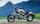The bus started from the village at speed 60 km/h. After one kilometer, when Milan came to bus stop on a motorcycle with grandmother. When they found that bus drove away he try to catch it. At the next stop, a distance of 3km caught up bus. How quickly had
2. Pedestrian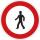Pedestrian came started at 8h in the morning with speed 4.4 km/h. At half-past eleven cyclist started at 26 km/h same way. How many minutes take cyclist to catch up pedestrian?
3. Two trains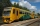From station A, the freight train traveled at a speed of 40 km/h in 9h. When he drove 15km, the fast train started from station A in the same direction at a speed of 70km/h. When will it the freight train catch up?
4. The ship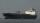The ship went upstream speed of 20 km/h in relation to water. River flows at speed 10 km/h. After half an hour, he stopped and returned downstream to the starting point. How long it took way back when even if downstream speed of ship is 20 km/h in relation
5. Cars 2Opava is 360 km distance from Prešov. At 8:00 hour two cars started against each other from this cities at average speeds 88 km/h and 121 km/h. At what hour its will meet and what distance its travel if car started from Prešov is faster?
6. Round-tripA woman works at a law firm in city A which is about 50 miles from city B. She must go to the law library in city B to get a document. Find how long it takes her to drive​ round-trip if she averages 40 mph.
7. EquationSolve the equation: 1/2-2/8 = 1/10; Write the result as a decimal number.
8. Cenda and Pepa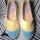Cenda and Pepa went to the event. Cenda started alone. Canda started after him for 20 minutes. How long took Cenda to catch him? Cenda traveling at 15 km/h, and Pepa traveling at 25 km/h.
9. Six yearsIn six years Jan will be twice as old as he was six years ago. How old is he?
10. Unknown numberIdentify unknown number which 1/5 is 40 greater than one tenth of that number.
11. Cars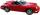Cars started at 7:00 AM. From point A car run at 40 km per hour, from B car run 70 km per hour. Between A and B is 250 km long road. When and where will meet (measured from point A)?
12. Fifth of the numberThe fifth of the number is by 24 less than that number. What is the number?
13. Cars 6At 9:00 am two cars started from the same town and traveled at a rate of 35 miles per hour and the other car traveled at a rate of 40 miles per hour. After how many hours will the cars be 30 miles apart?
14. CandyPeter had a sachet of candy. He wanted to share with his friends. If he gave them 30 candies, he would have 62 candies. If he gave them 40 candies, he would miss 8 candies. How many friends did Peter have?
15. Simple equationSolve for x: 3(x + 2) = x - 18
16. StormSo far, a storm has traveled 35 miles in 1/2 hour in direction straight to observer. If it is currently 5:00 p. M. And the storm is 105 miles away from you, at what time will the storm reach you? Explain how you solved the problem.
17. Father and sonFather is 48 years old, son 23. When will be the father twice older than a son?